How Cheenta works to ensure student success?
Explore the Back-Story

# Test of Mathematics Solution Subjective 110 - Ratio of Diagonals of Cyclic QuadrilateralThis is a Test of Mathematics Solution Subjective 110 (from ISI Entrance). The book, Test of Mathematics at 10+2 Level is Published by East West Press. This problem book is indispensable for the preparation of I.S.I. B.Stat and B.Math Entrance.

Also visit: I.S.I. & C.M.I. Entrance Course of Cheenta

## Problem:

:
Let ABCD be a cyclic quadrilateral with lengths of sides AB = p , BC = q , CD = r, and DA = s . Show that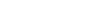## Solution:

Since ABCD is cyclic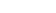is similar to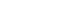(since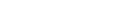as they are subtended by the same arc AD, andas vertically opposite angles are equal)

Hence their corresponding sides are proportional.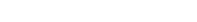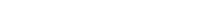Similarly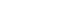is similar to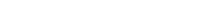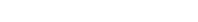Hence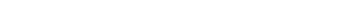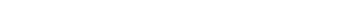Hence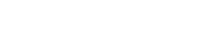Finally we note that sinceis similar to.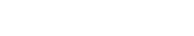This proves that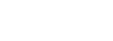This is a Test of Mathematics Solution Subjective 110 (from ISI Entrance). The book, Test of Mathematics at 10+2 Level is Published by East West Press. This problem book is indispensable for the preparation of I.S.I. B.Stat and B.Math Entrance.

Also visit: I.S.I. & C.M.I. Entrance Course of Cheenta

## Problem:

:
Let ABCD be a cyclic quadrilateral with lengths of sides AB = p , BC = q , CD = r, and DA = s . Show that## Solution:

Since ABCD is cyclicis similar to(sinceas they are subtended by the same arc AD, andas vertically opposite angles are equal)

Hence their corresponding sides are proportional.Similarlyis similar toHenceHenceFinally we note that sinceis similar to.This proves that### Knowledge Partner